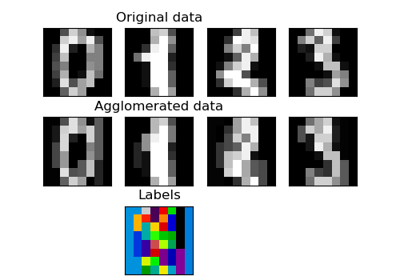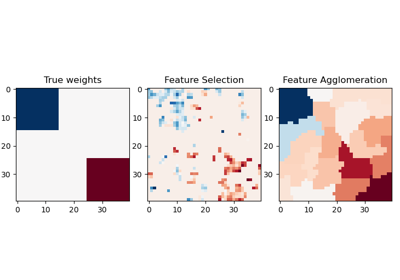# sklearn.cluster.FeatureAgglomeration¶

class sklearn.cluster.FeatureAgglomeration(n_clusters=2, *, affinity='euclidean', memory=None, connectivity=None, compute_full_tree='auto', linkage='ward', pooling_func=<function mean>, distance_threshold=None)[source]

Agglomerate features.

Similar to AgglomerativeClustering, but recursively merges features instead of samples.

Read more in the User Guide.

Parameters
n_clustersint, default=2

The number of clusters to find. It must be None if distance_threshold is not None.

affinitystr or callable, default=’euclidean’

Metric used to compute the linkage. Can be “euclidean”, “l1”, “l2”, “manhattan”, “cosine”, or ‘precomputed’. If linkage is “ward”, only “euclidean” is accepted.

memorystr or object with the joblib.Memory interface, default=None

Used to cache the output of the computation of the tree. By default, no caching is done. If a string is given, it is the path to the caching directory.

connectivityarray-like or callable, default=None

Connectivity matrix. Defines for each feature the neighboring features following a given structure of the data. This can be a connectivity matrix itself or a callable that transforms the data into a connectivity matrix, such as derived from kneighbors_graph. Default is None, i.e, the hierarchical clustering algorithm is unstructured.

compute_full_tree‘auto’ or bool, optional, default=’auto’

Stop early the construction of the tree at n_clusters. This is useful to decrease computation time if the number of clusters is not small compared to the number of features. This option is useful only when specifying a connectivity matrix. Note also that when varying the number of clusters and using caching, it may be advantageous to compute the full tree. It must be True if distance_threshold is not None. By default compute_full_tree is “auto”, which is equivalent to True when distance_threshold is not None or that n_clusters is inferior to the maximum between 100 or 0.02 * n_samples. Otherwise, “auto” is equivalent to False.

Which linkage criterion to use. The linkage criterion determines which distance to use between sets of features. The algorithm will merge the pairs of cluster that minimize this criterion.

• ward minimizes the variance of the clusters being merged.

• average uses the average of the distances of each feature of the two sets.

• complete or maximum linkage uses the maximum distances between all features of the two sets.

• single uses the minimum of the distances between all observations of the two sets.

pooling_funccallable, default=np.mean

This combines the values of agglomerated features into a single value, and should accept an array of shape [M, N] and the keyword argument axis=1, and reduce it to an array of size [M].

distance_thresholdfloat, default=None

The linkage distance threshold above which, clusters will not be merged. If not None, n_clusters must be None and compute_full_tree must be True.

New in version 0.21.

Attributes
n_clusters_int

The number of clusters found by the algorithm. If distance_threshold=None, it will be equal to the given n_clusters.

labels_array-like of (n_features,)

cluster labels for each feature.

n_leaves_int

Number of leaves in the hierarchical tree.

n_connected_components_int

The estimated number of connected components in the graph.

New in version 0.21: n_connected_components_ was added to replace n_components_.

children_array-like of shape (n_nodes-1, 2)

The children of each non-leaf node. Values less than n_features correspond to leaves of the tree which are the original samples. A node i greater than or equal to n_features is a non-leaf node and has children children_[i - n_features]. Alternatively at the i-th iteration, children[i] and children[i] are merged to form node n_features + i

distances_array-like of shape (n_nodes-1,)

Distances between nodes in the corresponding place in children_. Only computed if distance_threshold is not None.

Examples

>>> import numpy as np
>>> from sklearn import datasets, cluster
>>> images = digits.images
>>> X = np.reshape(images, (len(images), -1))
>>> agglo = cluster.FeatureAgglomeration(n_clusters=32)
>>> agglo.fit(X)
FeatureAgglomeration(n_clusters=32)
>>> X_reduced = agglo.transform(X)
>>> X_reduced.shape
(1797, 32)


Methods

 fit(X[, y]) Fit the hierarchical clustering on the data fit_transform(X[, y]) Fit to data, then transform it. get_params([deep]) Get parameters for this estimator. Inverse the transformation. set_params(**params) Set the parameters of this estimator. Transform a new matrix using the built clustering
__init__(n_clusters=2, *, affinity='euclidean', memory=None, connectivity=None, compute_full_tree='auto', linkage='ward', pooling_func=<function mean>, distance_threshold=None)[source]

Initialize self. See help(type(self)) for accurate signature.

fit(X, y=None, **params)[source]

Fit the hierarchical clustering on the data

Parameters
Xarray-like of shape (n_samples, n_features)

The data

yIgnored
Returns
self
property fit_predict

Fit the hierarchical clustering from features or distance matrix, and return cluster labels.

Parameters
Xarray-like, shape (n_samples, n_features) or (n_samples, n_samples)

Training instances to cluster, or distances between instances if affinity='precomputed'.

yIgnored

Not used, present here for API consistency by convention.

Returns
labelsndarray, shape (n_samples,)

Cluster labels.

fit_transform(X, y=None, **fit_params)[source]

Fit to data, then transform it.

Fits transformer to X and y with optional parameters fit_params and returns a transformed version of X.

Parameters
X{array-like, sparse matrix, dataframe} of shape (n_samples, n_features)
yndarray of shape (n_samples,), default=None

Target values.

**fit_paramsdict

Returns
X_newndarray array of shape (n_samples, n_features_new)

Transformed array.

get_params(deep=True)[source]

Get parameters for this estimator.

Parameters
deepbool, default=True

If True, will return the parameters for this estimator and contained subobjects that are estimators.

Returns
paramsmapping of string to any

Parameter names mapped to their values.

inverse_transform(Xred)[source]

Inverse the transformation. Return a vector of size nb_features with the values of Xred assigned to each group of features

Parameters
Xredarray-like of shape (n_samples, n_clusters) or (n_clusters,)

The values to be assigned to each cluster of samples

Returns
Xarray, shape=[n_samples, n_features] or [n_features]

A vector of size n_samples with the values of Xred assigned to each of the cluster of samples.

set_params(**params)[source]

Set the parameters of this estimator.

The method works on simple estimators as well as on nested objects (such as pipelines). The latter have parameters of the form <component>__<parameter> so that it’s possible to update each component of a nested object.

Parameters
**paramsdict

Estimator parameters.

Returns
selfobject

Estimator instance.

transform(X)[source]

Transform a new matrix using the built clustering

Parameters
Xarray-like of shape (n_samples, n_features) or (n_samples,)

A M by N array of M observations in N dimensions or a length M array of M one-dimensional observations.

Returns
Yarray, shape = [n_samples, n_clusters] or [n_clusters]

The pooled values for each feature cluster.

## Examples using sklearn.cluster.FeatureAgglomeration¶